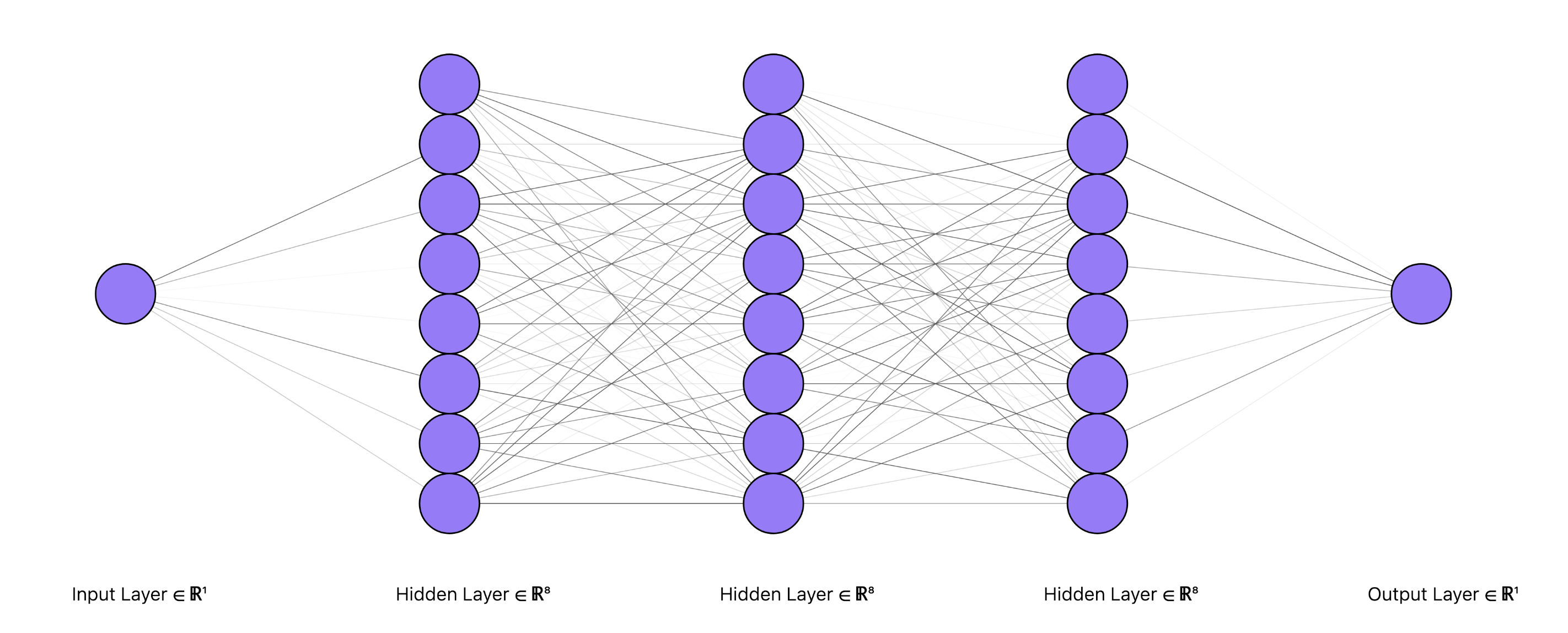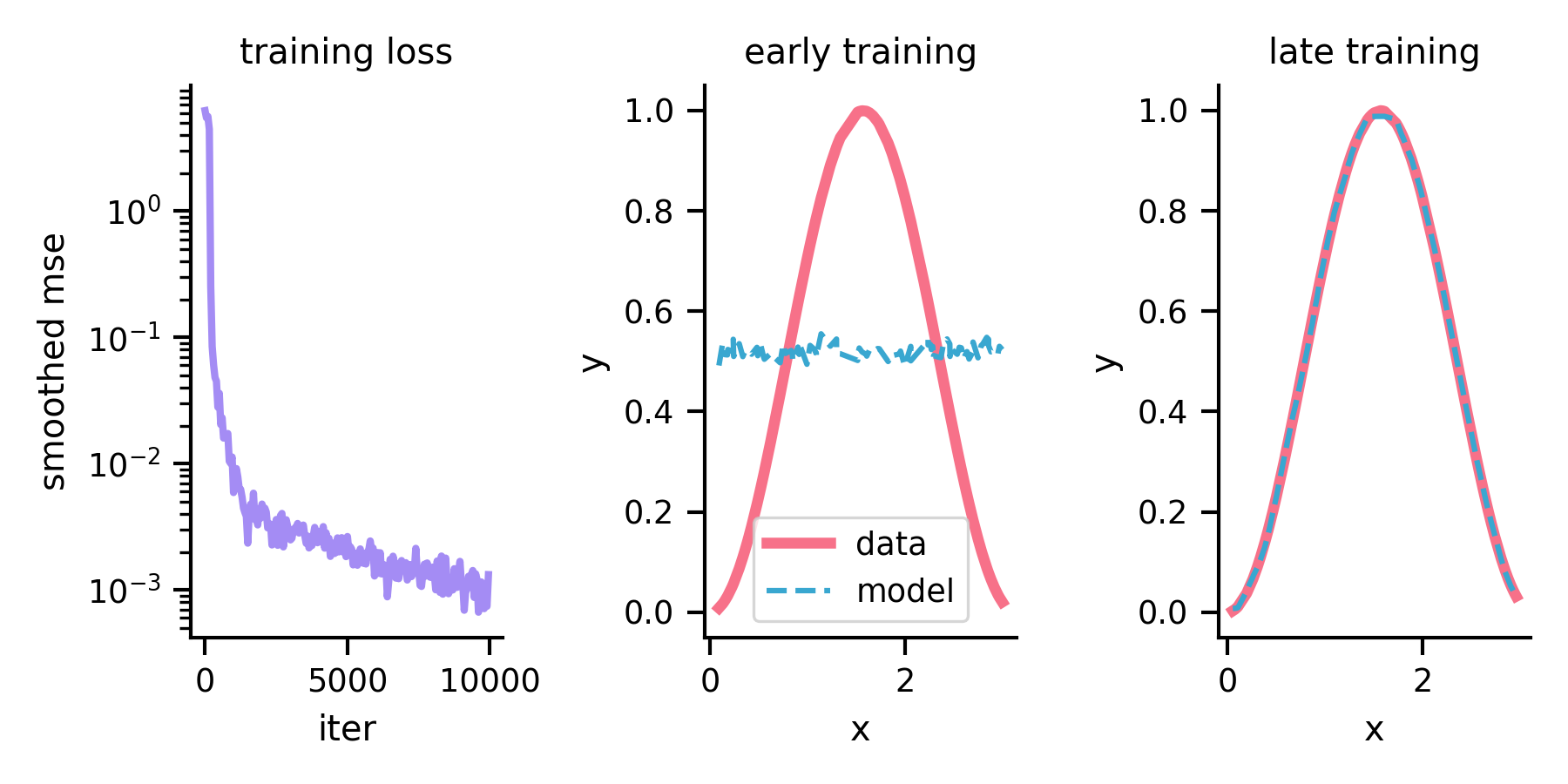# C++ neural networks from scratch – Pt 3. model training

Training a multilayer perceptron built in pure C++.## Fitting a neural network to data

We’ve built a tiny matrix library, and a flexible multilayer perceptron (MLP) with forward and backward methods. Now, it’s time to test if it can learn and fit data!Using the make_model() function (defined in Part 2) to create an MLP with 3 hidden layers with 8 hidden units each, we just need to write code for the data generation and the model training loop.

// main.cpp
#include "matrix.h" // contains matrix library
#include "nn.h"  // contains our MLP implementation

int main() {

// init model
int in_channels{1}, out_channels{1};
int hidden_units_per_layer{8}, hidden_layers{3};
float lr{.5f};

auto model = make_model(
in_channels,
out_channels,
hidden_units_per_layer,
hidden_layers,
lr);

// open file to save loss, x, y, and model(x)
std::ofstream my_file;
my_file.open ("data.txt");

int max_iter{10000};
float mse;

//////////////////////////////////
////* training loop goes here*////
//////////////////////////////////

my_file.close();
}


## Writing the training loop

Let’s fit our model to a nonlinear function: $$y = \sin^2(x)$$ where $$x\in[0, \pi)$$. On each iteration, we’ll generate an (x, y) pair using this function, then pass x through our model, $$\hat{y} \leftarrow \texttt{model}(x)$$, and use our model.backprop() method to compute the gradient and backpropagate with respect to $$\texttt{loss}\leftarrow (y-\hat{y})^2$$.

/* training loop */
const float PI {3.14159};
for(int i = 1; i<=max_iter; ++i) {

// generate (x, y) training data: y = sin^2(x)
auto x = mtx<float>::rand(in_channels, 1).multiply_scalar(PI);
auto y = x.apply_function([](float v) -> float { return sin(v) * sin(v); });

// forward and backward
auto y_hat = model.forward(x);
model.backprop(y); // loss and grads computed in here

// function that logs (loss, x, y, y_hat)
log(file, x, y, y_hat);
}


## Trained model

I logged the (loss, x, y, y_hat) values to a .txt file, then parsed & plotted them in Python (plotting code is also included in the repo). The model clearly learns the function and reduces error over time (left panel). This is also qualitatively evident comparing the model outputs toward the beginning of training (middle panel – looks like trash) to those from the late phase of training (right panel). Looks pretty great :).## Recap

We’ve come a long way: from zero to a fully trained model in just a couple hundred lines of C++. We built our own Matrix class with linear algebra capabilities, and a flexible implementation of a backprop-able MLP. Despite this being a relatively simple task in, say, Python, it’s fun to do away with all the machine learning library abstractions and just write things yourself – back to basics. I find that in higher-level languages you’re always worrying about whether or not your implementation is as efficient as it could be. E.g. when writing loops in some analysis code you always wonder if there’s a better vectorized approach. In C++. since you’re just so close to the bare metal, it seems to me like you just… write the loops. Julia kind of has the same vibes but C++ feels a bit more satisfyingly raw.

This was my very first C++ project, and it covered a lot of useful topics to get familiarized with the language: strict typing & type inference, OOP, the standard library, and templated programming are a few things that immediately come to mind. I’m a long way to writing fully idiomatic C++ (the code written in this project has a Pythonic flavour to it) but it’ll be fun to look back at this down the road and see how I’ve improved.

tags:

updated: